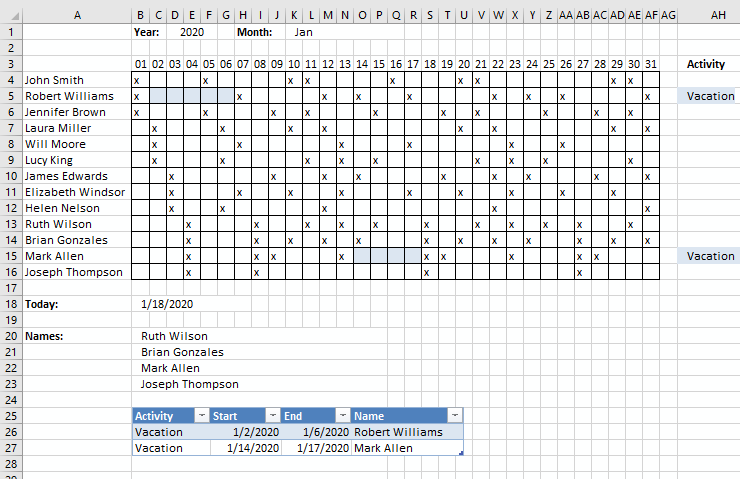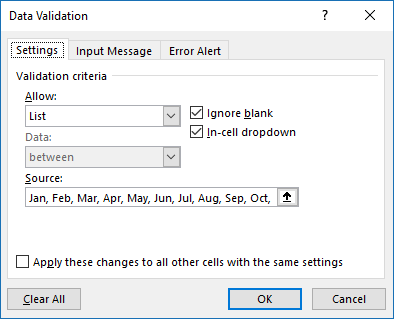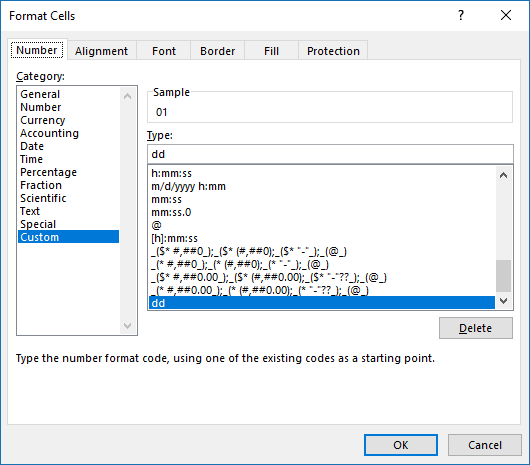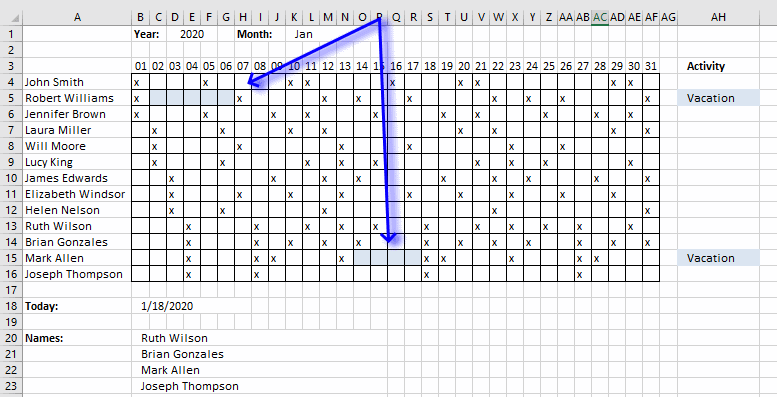Author: Oscar Cronquist Article last updated on April 10, 2019This schedule uses the year and month in cell D1 and K1 to highlight activities like vacation specified in the Excel defined Table. The schedule works like this, enter a date in cell B18 and a formula in cell B20 extracts the names that have an x for the given date.

The names and x are entered manually, however, the activity cells are highlighted automatically based on the start and end date specified in the Excel defined Table.

The date calculations in row 3 are based on the selected year and month, you don't need to change these dates. The activity column AH is based on the name of the activity, start, end date, and the name in the Excel defined Table.

### How I built this schedule

#### Date drop-down list

Cell K1 contains a drop-down list that lets you pick a month, here is how I created it:

1. Select cell K1.
2. Press with left mouse button on tab "Data" on the ribbon.
3. Press with left mouse button on "Data Validation" button.4. Choose "List".
5. Type "Jan, Feb, Mar, Apr, May, Jun, Jul, Aug, Sep, Oct, Nov, Dec" without double quotes in Source:.
6. Press with left mouse button on OK button.

#### Dates in row 3

These dates are dynamic meaning they change depending on what year and month the user have selected.

Formula in cell B3:

=DATE(D1, MATCH(K1, {"Jan", "Feb", "Mar", "Apr", "May", "Jun", "Jul", "Aug", "Sep", "Oct", "Nov", "Dec"}, 0), 1)

#### Explaining formula in cell B3

We need to create an Excel date and to do that we have the DATE function. It needs three values, year, month, and day. The month value is a number between 1 and 12. 1 is January is 1, February is 2 and March is 3, etc.

Step 1 - Convert month name to the numerical equivalent

MATCH(K1, {"Jan", "Feb", "Mar", "Apr", "May", "Jun", "Jul", "Aug", "Sep", "Oct", "Nov", "Dec"}, 0)

becomes

MATCH("Jan", {"Jan", "Feb", "Mar", "Apr", "May", "Jun", "Jul", "Aug", "Sep", "Oct", "Nov", "Dec"}, 0)

and returns 1. This means that "Jan" has position 1 in the array. "Jan" is also the first month in a year.

Step 2 - Create Excel date

DATE(D1, MATCH(K1, {"Jan", "Feb", "Mar", "Apr", "May", "Jun", "Jul", "Aug", "Sep", "Oct", "Nov", "Dec"}, 0), 1)

becomes

DATE(D1, 1, 1)

becomes

DATE(2020, 1, 1)

and returns 43831. Excel formats the number as an Excel date and shows only 01 in cell B3.

Formula in cell C3:

=B3+1

This formula is copied to cell range D3:AF3.

Select cell range B3:AH3. Press CTRL + 1 to format cells.Press with left mouse button on "Custom" and then use type dd to only show the day number. Press with left mouse button on OK button to apply changes.

#### Conditional formattingI use conditional formatting to highlight specific date ranges based on the Excel defined Table.

1. Select cell range B4:AF16.
2. Go to tab "Home" on the ribbon.
3. Press with left mouse button on "Conditional Formatting" button.
4. Press with left mouse button on "New Rule...".
5. Press with left mouse button on "Use a formula to determine which cells to format".
6. Type the following formula:
=COUNTIFS(INDIRECT("Table1[Start]"), "<="&B\$3,INDIRECT("Table1[End]"), ">="&B\$3, INDIRECT("Table1[Name]"), \$A4)
7. Press with left mouse button on "Format..." button.
8. Go to tab "Fill".
9. Pick a color.
10. Press with left mouse button on OK button.
11. Press with left mouse button on OK button.

#### Explaining Conditional formatting formula in cell B4

The COUNTIFS function counts the number of rows in the Excel defined Table that meets three conditions. Date =< Start, Date =>End, and if name equal to a name in the table. If all three are met then the formula returns 1 and the cell is highlighted.

COUNTIFS(criteria_range1, criteria1, [criteria_range2, criteria2], [criteria_range3, criteria3]…)

The formula also contains cell references that change when the CF moves to the next cell, you can read more about it here: How to use absolute and relative references

Step 1 - Is date in cell B3 larger than or equal to start dates?

criteria_range1 is a cell reference or structured reference to Table1 and column Start. Excel is not happy with structured references in Conditional formatting formulas, however, there is a workaround. Simply use the INDIRECT function and Excel is happy again.

INDIRECT("Table1[Start]")

criteria1 is a cell reference to the date in cell B3. The <= is logical operators that make the COUNTIFS function check that the date in B3 is larger or equal to the dates in Table1[Start]. The ampersand character & concatenates the logical operators and the date in cell B3. The double quotes are needed in order to treat the logical operators as text.

"<="&B\$3

Note, the cell references changes to the next cell C\$3 when the CF formula moves.

INDIRECT("Table1[Start]"), "<="&B\$3

returns

{43832;43844}, "<=43831"

Step 2 - Is date in cell B3 smaller than or equal to end dates?

Here is criteria_range2 and criteria2.

INDIRECT("Table1[End]"), ">="&B\$3

returns

{43836;43847}, ">=43831"

Step 3 - Is name in cell \$A4 equal to name in Table1?

INDIRECT("Table1[Name]"), \$A4

returns

{"Robert Williams";"Mark Allen"}, "John Smith"

Step 4 - All conditions together

COUNTIFS(INDIRECT("Table1[Start]"), "<="&B\$3,INDIRECT("Table1[End]"), ">="&B\$3, INDIRECT("Table1[Name]"), \$A4)

becomes

COUNTIFS({43832;43844}, "<=43831",{43836;43847}, ">=43831", {"Robert Williams";"Mark Allen"}, "John Smith")

and returns 0 (zero). Cell B4 is not highlighted.

#### Formula in cell AH4

This formula checks if the name and the corresponding start and end dates overlap the selected year and month. It returns the activity name if conditions are met.

=IFERROR(INDEX(Table1[Activity], SMALL(IF((A4=Table1[Name])*(Table1[Start]>=DATE(\$D\$1, MATCH(\$K\$1, {"Jan", "Feb", "Mar", "Apr", "May", "Jun", "Jul", "Aug", "Sep", "Oct", "Nov", "Dec"}, 0), 1))*(Table1[End]<=DATE(\$D\$1, MATCH(\$K\$1, {"Jan", "Feb", "Mar", "Apr", "May", "Jun", "Jul", "Aug", "Sep", "Oct", "Nov", "Dec"}, 0)+1, 1)-1), MATCH(ROW(Table1[Activity]), ROW(Table1[Activity])), ""), 1)), "")

#### Formula in cell B20

You can find an explanation of this formula in the following article: Shift Schedule

### Get the Excel fileWatch-schedule-that-populates-vacation-time.xlsx